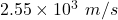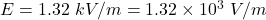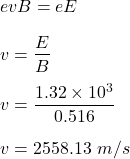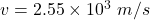## An electric field of 1.32 kV/m and a magnetic field of 0.516 T act on a moving electron to produce no net force. If the fields are perpendic

Question

An electric field of 1.32 kV/m and a magnetic field of 0.516 T act on a moving electron to produce no net force. If the fields are perpendicular to each other, what is the electron’s speed?

in progress 0
2 weeks 2021-08-28T18:08:48+00:00 1 Answers 0 views 0

The speed of the electron is.

Explanation:

Given that,

The magnitude of electric field,The magnitude of magnetic field, B = 0.516 T

Both the magnetic and electric fields are acting on the moving electron. Then,  the magnitude of electric field and magnetic field is balanced such that :orSo, the speed of the electron is. Hence, this is the required solution.